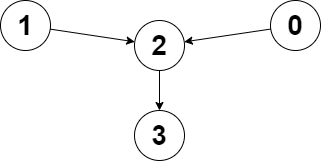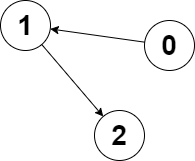# 2359. Find Closest Node to Given Two Nodes

Medium
You are given a directed graph of `n` nodes numbered from `0` to `n - 1`, where each node has at most one outgoing edge.
The graph is represented with a given 0-indexed array `edges` of size `n`, indicating that there is a directed edge from node `i` to node `edges[i]`. If there is no outgoing edge from `i`, then `edges[i] == -1`.
You are also given two integers `node1` and `node2`.
Return the index of the node that can be reached from both `node1` and `node2`, such that the maximum between the distance from `node1` to that node, and from `node2` to that node is minimized. If there are multiple answers, return the node with the smallest index, and if no possible answer exists, return `-1`.
Note that `edges` may contain cycles.
Example 1:Input: edges = [2,2,3,-1], node1 = 0, node2 = 1
Output: 2
Explanation: The distance from node 0 to node 2 is 1, and the distance from node 1 to node 2 is 1.
The maximum of those two distances is 1. It can be proven that we cannot get a node with a smaller maximum distance than 1, so we return node 2.
Example 2:Input: edges = [1,2,-1], node1 = 0, node2 = 2
Output: 2
Explanation: The distance from node 0 to node 2 is 2, and the distance from node 2 to itself is 0.
The maximum of those two distances is 2. It can be proven that we cannot get a node with a smaller maximum distance than 2, so we return node 2.
Constraints:
• `n == edges.length`
• `2 <= n <= 10^5`
• `-1 <= edges[i] < n`
• `edges[i] != i`
• `0 <= node1, node2 < n`

### 解題

func closestMeetingNode(edges []int, node1 int, node2 int) int {
var bfs func(int) []int
bfs = func(node int) []int {
stack := []int{ node }
l := make([]int, len(edges)) // 記錄 node 可以到的地方需要的步數
l[node] = 0
visited := make([]bool, len(edges)) // 記錄走過的點，不需要再加入 stack 了
visited[node] = true
for len(stack) != 0 {
cur := stack // 目前走到的點
stack = stack[1:] // 把目前走到的點從 stack 移除
nxt := edges[cur] // 下一個可以到的點
if nxt == -1 || visited[nxt] { // 接下來沒點可以走了，或是下一個點走過了
break
} else {
l[nxt] = l[cur] + 1
stack = append(stack, nxt)
visited[nxt] = true
}
}
return l
}
l1 := bfs(node1) // 記錄 node1 到每個點需要的步數
l2 := bfs(node2) // 記錄 node2 到每個點需要的步數
ans := -1 // 答案
tmp := math.MaxInt32 // 目前到答案節點需要的步數
for i:=0; i<len(edges); i++ {
if l1[i] == 0 && i != node1 { continue } // 非 node1 、到達的步數卻是0，代表這個點 node1 根本到不了
if l2[i] == 0 && i != node2 { continue } // 非 node2 、到達的步數卻是0，代表這個點 node2 根本到不了
if max(l1[i], l2[i]) < tmp { // 兩個點都可以到達 i ，且步數比到目前的答案更少
tmp = max(l1[i], l2[i]) // 更新步數
ans = i // 更新答案
}
}
return ans
}
func max(a, b int) int {
if a > b {
return a
}
return b
}